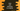# Different ways to add elements to an ArrayList in Java## How to add elements to an ArrayList in Java:

Java ArrayList provides a method called add that can be used to add elements to the ArrayList. This method is used to append an element to the end of the ArrayList or we can use it to add the element at any specific position.

Let’s learn how to use this with examples.

### Append an element to the end of the ArrayList:

The add method is defined as:

``public boolean add(E e)``

Where, e is the element to append to the ArrayList. It will append the element to the end of the ArrayList and return true.

Let’s try it with an example:

``````import java.util.ArrayList;

public class Main {
public static void main(String[] args) {
ArrayList<Integer> givenList = new ArrayList<>();

System.out.println(givenList);
}
}``````

In this example, we created an empty ArrayList and it used add() to append three numbers to the ArrayList. If you run this program, it will print the below output:

### Example 2: Append an element at any specific position:

We can use the add method to append an element at any specific position. Following is the method definition:

``public void add(int index, E e)``

Here,

• index is the index at which the element needs to be inserted.
• e is the element to insert.

Note that this method will throw IndexOutOfBoundsException if the given index is invalid. For example, if we pass a negative index or any value that is greater than the size of the ArrayList, it will throw IndexOutOfBoundsException.

Let’s try it with an example:

``````import java.util.ArrayList;

public class Main {
public static void main(String[] args) {
ArrayList<Integer> givenList = new ArrayList<>();

``[0, 1, 2, 3]``